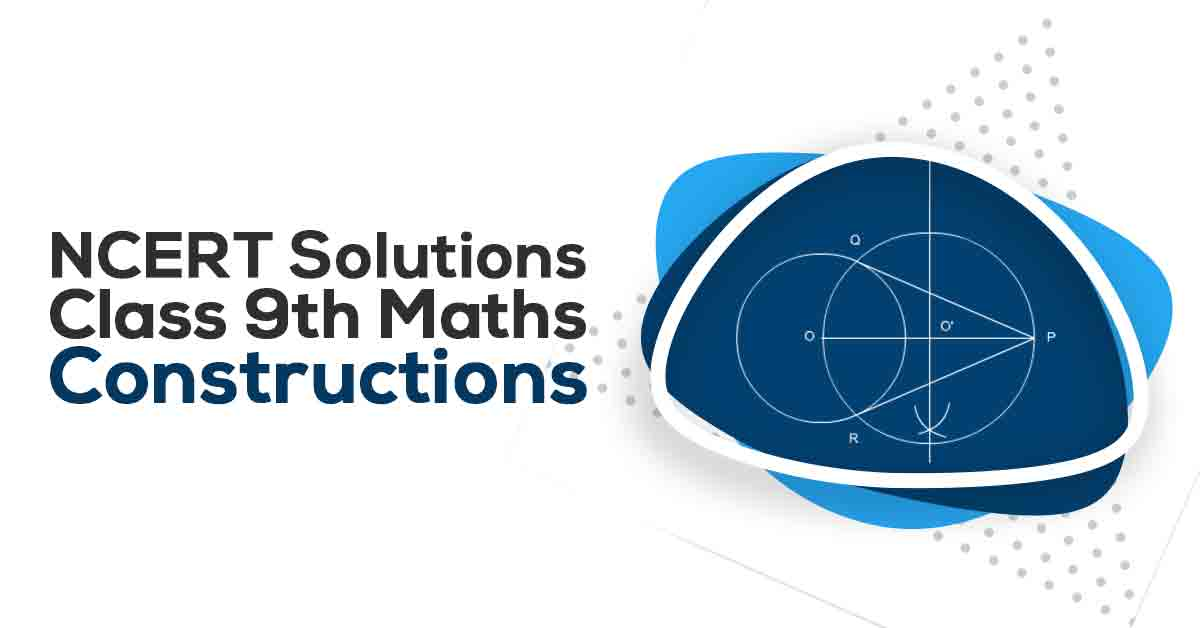Class 9th Math Contructions NCERT Solution CBSE 2023

# NCERT Solutions For Class 9 Maths Chapter 11

## NCERT Solutions For Class 9 Maths Chapter 11 Constructions - PDF DownloadStudents find NCERT Solutions for Class 9 Maths Chapter 11 Constructions quite helpful for their Exam Preparation. In this chapter, you will learn to bisect a given angle.  To draw the perpendicular bisector of a given line segment.  To construct an angle of 60° etc. To construct a triangle given its base, a base angle and the sum of the other two sides.  To construct a triangle given its base, a base angle and the difference of the other two sides.

These CBSE NCERT Solutions for Class 9 Maths Chapter 11 Constructions will help you to practice all the topics in the chapter with ease and score maximum marks in the examination.